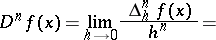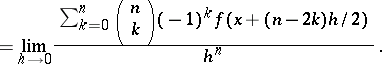# Schwarz symmetric derivative

of a functionat a pointIt is sometimes called the Riemann derivative or the second symmetric derivative. For the first time introduced by B. Riemann in 1854 (see ); it was studied by H.A. Schwarz . More generally, the symmetric derivative of orderis also called a Schwarz symmetric derivative: